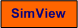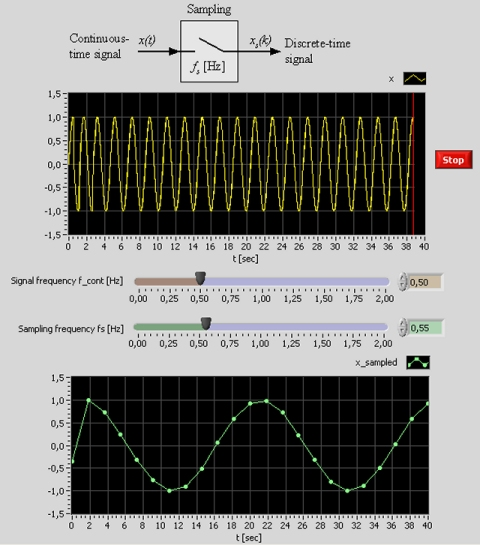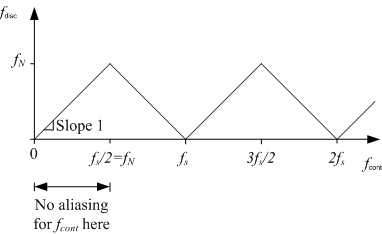# Aliasing at Sampling

Snapshot of the front panel of the simulator:• What is needed to run the the simulator? Read to get most recent information!
• Tips for using the simulator.
• The simulator: . The simulator runs immediately after the download by clicking Open in the download window. Alternatively, you can first save a copy of the exe-file on any directory (folder) on your PC and then run the exe-file, which starts the simulator.

## Description of the system to be simulated

Sampling of sinusoid, x(t), is simulated. The sampling results the discrete-time signal xs(k) where k is a time index (number of samples). Both signals are plotted simultaneously. You can adjust both the signal frequency and the signal frequency.

## Aim

The aim is to develop an understanding of the aliasing phenomenon.

## Motivation

Sampling av continuous-time signals takes place in all applications where a computer is used to read measurement data. If the sampling rate is too low compared to the frequency of the signal to be sampled, aliasing occurs. Aliasing means that the discrete-time signal gets a lower frequency than the original signal. Obviously, sampling can cause problems in applications, e.g. in audio applications.

## Theory

Given a continuous-time signal, x(t), of frequency fcont [Hz] which is sampled with sampling frequency fs [Hz]. The Nyquist frequency fN is defined as half of the sampling frequency:

fN = fs/2

It ca be shown that if the signal frequency fcont is larger than fN, there is aliasing, which implies that the resulting discrete-time signal, xs, gets a frequency, fdisc, which is smaller than the signal frequency fcont. Figure 1 shows the relation between fdisc and fcont.Figure 1

From the figure one observation is that fdisc is equal to the difference fsfcont if  fcont is between fN and fs.